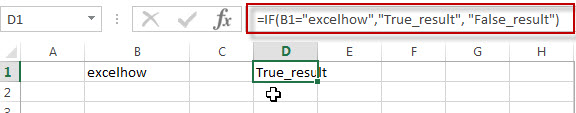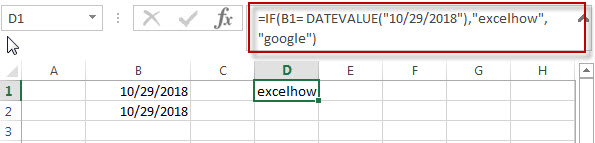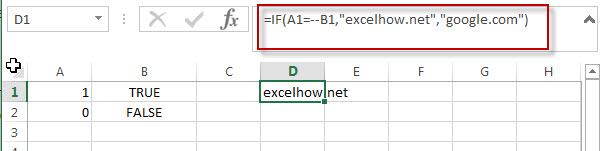# Excel IF formula with “Equal to” logical operators

The “Equal to” logical operator can be used to compare the below data types, such as: text string, numbers, dates, Booleans.  This section will guide you how to use “equal to” logical operator in excel IF formula with text string value and dates value.

 Formula example Description =IF(A1=B1,“TRUE”,”FALSE”) Returns TRUE if a value in cell A1 is equal to the value in cell B1, FALSE if not. =IF(A1=”excehow”,”TRUE”,”FALSE”) Returns TRUE if a value in cell A1 is equal to “excelhow”, FALSE if not. =IF(A1=TRUE,”TRUE”,”FALSE”) Returns TRUE if a value in cell A1 is equal to TRUE, FALSE if not. =IF(A1=(B1/5),”TRUE”,”FALSE”) Returns TRUE if a value in cell A1 is equal to the quotient of the division of B1 by 5, FALSE if not.

## The Generic IF formula using “equal to” logical operators with text values

Let’ see the below formula:

`=IF(B1=”excelhow”,True_result, False_result)`If you want to do some special action when a value in a cell equal to a certain value, then you can use IF function with logical operator to constructing a condition statement. If the condition statement is true, then do some action.

The logical test is B1=”excelhow”in the above formula, and if cell B1 is equal to the string “excelhow”, then returns “True_results“； If FALSE, return “False_result”.

We want to set the value in Cell D1, we only need to take action when the condition test is TRUE. Here, we will add“True_result” string to Cell D1 when the value in cell B1 is “excelhow”. If the value in cell B1 is not “excelhow”, then we will add “False_result” string into Cell D1.

## The Generic IF formula using “equal to “   logical operators with dates value

If the cells B1 and B2 contain the date value “10/29/2018”, if we use logic test B1=”10/29/2018” in the formula =B1=”10/29/2018”, you will get the FALSE result. Or if you use logic test B1=B2 in formula =B1=B2, then it will return TRUE.

So if you want to check one value in cells, you must use DATEVALUE function to warp a date to compare with a specific date value. For example: =B1=DATEVALUE(“10/29/2018”), at this time, it will return TRUE.

Now we can write a Generic IF formula using “equal to” with date value as follows:

`=IF(B1= DATEVALUE(“10/29/2018”),”excelhow”,”google”)`## The Generic IF formula using “equal to “   logical operators with Boolean values and numbers

The Boolean value of TRUE Values equates to 1 and The FALSE value is equal to 0.

When writing a logical test using “equal to” operator that compares a Boolean value and a number. Assuming that using the logical test “1=True”, you will get the False result. it’s not our expected result.  At this time, you can use the double minus sign in the front of a Boolean value in a logical test. For example:”1=–TRUE

`=IF(A1=--B1,"excelhow.net","google.com")`### Related Formulas

• Excel IF formula with operator : greater than,less than
how to use if function with “greater than”,  “greater than or equal to”, ”less than” and “less than or equal to” logical operators in excel.Let’s see the below generic if formula with greater than operator:=IF(A1>10,”excelhow.net”,”google”) …

### Related Functions

• Excel IF function
The Excel IF function perform a logical test to return one value if the condition is TRUE and return another value if the condition is FALSE….
• Excel nested if function
The nested IF function is formed by multiple if statements within one Excel if function. This excel nested if statement makes it possible for a single formula to take multiple actions…